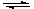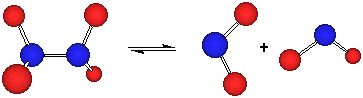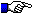Safety Emporium for all your lab and safety needs

# The General Chemistry Demo Lab

## P and V Effects on N2O4 Equilibrium

[View demo]   [How it works]

### Introduction

Le Chatlier's principle allows us to predict the direction a reaction will take when we perturb the equilibrium by changing the pressure, volume, temperature, or component concentrations.

Simply stated, the principle says that if an external stress is applied to a system at equilibrium, the system will adjust itself to minimize that stress.

A good non-chemical analogy is two people on a see-saw. If their masses are equal then the see-saw balances. If we stress the system by adding weight to one side, the only way we can return to balance is by having the heavier person move closer to the fulcrum.

For a general equilibrium such as this:

A + BC + D

If we add more of compound A to the system, the system will try to get rid of the excess A. The only way it can do that is to make the forward reaction (i.e. left to right) favored, producing more C and D as some of the excess A is consumed. Notice we can't convert all the excess A to C and D because the reverse reaction tries to get rid of the "new" C and D in the same fashion.

Likewise, if we took away some A, the system would try to make more A by consuming C and D (favoring the reverse reaction, i.e. from right to left).

Remember from the ideal gas law, PV=nRT, that pressure and volume are inversely related. If we change the pressure or volume of a gas, we are changing its concentration. Therefore, if we change P or V, the system is no longer at equilibrium and Le Chatlier's principle will apply.

Let's consider what happens when we increase the pressure on this reaction, something that we can also do by decreasing the volume

N2O4 (g)2 NO2 (g)

In the forward reaction, the N-N bond of N2O4 breaks and the two NO2 pieces fly apart in space. To go in the opposite direction, two NO2 molecules must not only come close together, but they also be aligned properly to form a new N-N bond. This will be important a little later, so keep it in mind.From Le Chatlier, we know that if we increase the pressure, the system will do what it can to counteract that change. Notice in this equation that the right side has two moles of gas but the left side has only one (n = +1). Therefore, one way to reduce the pressure would be to convert some of the NO2 (g) to N2O4 (g). In other words, we predict the reaction will shift to the left under these conditions.

We could express this in terms of the reaction quotient, Qc if we wished:

QC = [NO2]2/[N2O4]

Notice that as both concentrations increase, the value of QC becomes larger (because the top one is squared). Therefore, Qc > Kc, meaning there is an excess of products over reactants. Therefore, the system will shift to the left (consume the excess products) until QC = KC.

Whatever method we use to analyze the problem, it should become obvious that an increase in pressure (or decrease in volume) favors the net reactions that DECREASES the TOTAL number of moles of gases.A reaction that has no net change in the number of moles of gases (n = 0) will be unaffected by P or V changes!Adding an inert (non-reactive) gas to the reaction mixture has no effect on the gaseous equilibrium even though the pressure is higher! Why? Suppose we add 400 moles of helium gas to our reaction vessel. Which side of the equation does that go on? Both -- it doesn't react! Therefore, both of these reactions haven = +1.

400 He(g) + N2O4(g)2 NO2(g) + 400 He(g)

N2O4(g)2 NO2(g)

In fact, notice that their KC and QC's are identical as well:

 [NO2]2[He]400 [NO2]2 KC   = ----------------- = ----------- [N2O4][He]400 [N2O4][Intro]   [How it works]

### The Demo

The syringe below is filled with an equilibrium mixture of N2O4 and NO2 gases. The NO2 is a brown gas and N2O4 is colorless. Therefore, we can monitor changes in their relative concentrations by examining how dark or light the gas mixture becomes upon changes in P and V.

N2O4 (g)2 NO2 (g)

colorless           brown

If you have Apple's (free) Quicktime installed, you can watch a color movie of the demonstration. This movie is 337 Kb in size, so it may take a moment to download if you have a slow Internet connection.

To view the movie, simply click on the picture below. You can move through the movie with the controls, and you can step through it frame by frame with the arrow keys:[Intro]   [View demo]

### How it works

When we compress the gas in the syringe (decrease the volume = increase the pressure), the gas becomes dark brown but then almost immediately lightens again (step through the movie with the arrow keys...it is a subtle effect). Why?

The answer lies in kinetics. It takes a few seconds to establish equilibrium in this system. If we compress the syringe with infinite speed, we have the same number of NO2 gas molecules, but now they are in a smaller volume. More molecules per unit volume = a high concentration or density. Therefore, the gas should appear darker and it does!

But left to equilibrate at this new volume (pressure) for a few seconds, the reaction shifts according to the principles discussed above. There are fewer gas molecules on the left side of our equilibrium equation, so some of the brown NO2 molecules dimerize to form colorless N2O4. That decreases the concentration of NO2, so the color fades.

That makes intuitive sense as well...for two NO2 molecules to come together and dimerize means they must be in close contact with each other. If our gas was very dilute (low pressure and/or high volume), two NO2 molecules would rarely encounter each other and the reaction equilibrium would lie very far to the right.

At the very moment when we release the gas in the syringe (expand the volume = decrease the pressure), the gas becomes much lighter but then immediately darkens slightly (again, step through the movie with the arrow keys). Why?

It's kinetics again. If we change the volume instantly, we have the same number of brown molecules, but in a much larger volume (lower pressure). There are far fewer brown NO2 molecules per unit volume, so the gas should appear lighter.

Within a few seconds, the gas darkens as the equilibrium is re-established. As described above, at a low pressure it is very hard to get two NO2 molecules together in (and in the correct orientation to form an N-N bond) because there are so few of them per unit volume. Therefore, any N2O4 molecule that dissociates stands little chance of reassembling itself.

### How this relates to other concepts

Consider Henry's Law, c = kP where c = the concentration of a gas dissolved in a liquid, k is a constant and P is the pressure of the gas over the solution. This is an equilibrium situation -- gas molecules are constantly entering and leaving the solution (at the same rate if we are at equilibrium).

But notice that if we forgot Henry's Law, we would still know that the concentration of a gas in a liquid increases with pressure by applying the principles we discovered in this demonstration!

Let's start by writing a chemical equation for our closed system, and use carbonated water as an example:

H2O (l) + CO2 (aq)H2O (l) + CO2 (g)

If we increase the pressure, the system will respond shifting this equilibrium to the side of the equation that has fewer (no) moles of gas, the left side. In other words, some CO2 must enter the solution! As we increase P, more CO2 must dissolve!